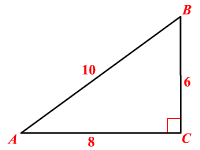# Sine

The sine of an angle is the trigonometric ratio of the opposite side to the hypotenuse of a right triangle containing that angle.

$\text{sine}=\frac{\text{length}\text{\hspace{0.17em}}\text{\hspace{0.17em}}\text{of}\text{\hspace{0.17em}}\text{\hspace{0.17em}}\text{the}\text{\hspace{0.17em}}\text{\hspace{0.17em}}\text{opposite}\text{\hspace{0.17em}}\text{\hspace{0.17em}}\text{to}\text{\hspace{0.17em}}\text{\hspace{0.17em}}\text{the}\text{\hspace{0.17em}}\text{\hspace{0.17em}}\text{angle}}{\text{length}\text{\hspace{0.17em}}\text{\hspace{0.17em}}\text{of}\text{\hspace{0.17em}}\text{\hspace{0.17em}}\text{the}\text{\hspace{0.17em}}\text{\hspace{0.17em}}\text{hypotenuse}}$ abbreviated as “sin”.

Example:

In the triangle shown, $\mathrm{sin}A=\frac{6}{10}\text{\hspace{0.17em}}\text{\hspace{0.17em}}\text{or}\text{\hspace{0.17em}}\text{\hspace{0.17em}}\frac{3}{5}\text{\hspace{0.17em}}\text{\hspace{0.17em}}\text{and}\text{\hspace{0.17em}}\text{\hspace{0.17em}}\mathrm{sin}B=\frac{8}{10}\text{\hspace{0.17em}}\text{\hspace{0.17em}}\text{or}\text{\hspace{0.17em}}\text{\hspace{0.17em}}\frac{4}{5}$ .The sine ratio is the same regardless of the size of the right triangle. So, it is often easiest to consider a right triangle with a hypotenuse of length $1$ .

The sine ratio can also be thought of as a function, which takes different values depending on the measure of the angle. You can measure an angle in degrees or  radians .

The reciprocal of the sine ratio is known as cosecant ratio of an angle, abbreviated as "cosec".

That is,

$\text{cosecant}=\frac{\text{length}\text{\hspace{0.17em}}\text{\hspace{0.17em}}\text{of}\text{\hspace{0.17em}}\text{\hspace{0.17em}}\text{the}\text{\hspace{0.17em}}\text{\hspace{0.17em}}\text{hypotenuse}}{\text{length}\text{\hspace{0.17em}}\text{\hspace{0.17em}}\text{of}\text{\hspace{0.17em}}\text{\hspace{0.17em}}\text{the}\text{\hspace{0.17em}}\text{\hspace{0.17em}}\text{leg}\text{\hspace{0.17em}}\text{\hspace{0.17em}}\text{opposite}\text{\hspace{0.17em}}\text{\hspace{0.17em}}\text{to}\text{\hspace{0.17em}}\text{\hspace{0.17em}}\text{the}\text{\hspace{0.17em}}\text{\hspace{0.17em}}\text{angle}}$

In the above example, $\text{cosec}\text{\hspace{0.17em}}A=\frac{9}{4}\text{\hspace{0.17em}}\text{\hspace{0.17em}}\text{and}\text{\hspace{0.17em}}\text{\hspace{0.17em}}\text{cosec}\text{\hspace{0.17em}}B=\frac{9}{7}$ .